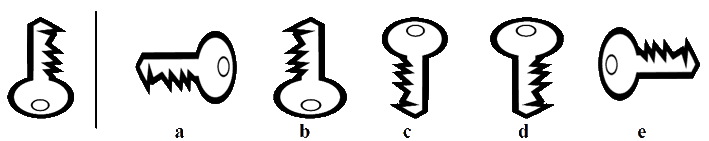# Type 3 - 'Rotations'

With this type of question you are given an image to the left of a vertical line. To the right of the vertical line you are given five more images. Four of these images are mirror images of the image to the left - One of these images can be rotated to form the image on the left.

You need to find which is the image that can be rotated to form the original.

Remember that the figures and diagrams consist of lines, shapes and shading/patterns. The shapes can shift in various ways. (rotate, reflect and transpose). The shapes can change in many ways. (increase/decrease, add and copy). They can consist of a movement, size or number sequence.Compare the original image on the left with each image on the right in turn and see if it would make the shape if rotated - take care, this is not as easy as it seems. Look for some detail of the image that is prominent - such as the jagged edge of the key in this case,

Image 'a' would need to be rotated 90° clockwise - but would have the jagged edges on the left.

Image 'b' needs no rotation - but the jagged edges are on the left.

Image 'c' would need to be rotated 180° - the jagged edges are on the right, the same as the original - looks like this is the correct one. Still check the others to make sure.

Image 'd' would need to be rotated 180° - but would have the jagged edges on the left.

Image 'e' needs to be rotated 90° anticlockwise - but would have the jagged edges on the left.

### So, image 'c' is the correct answer.

If you are having problems visualising this, print out the questions, cut out the image and rotate it physically,

Practice working with Non Verbal Reasoning Type 3 questions with this
Non Verbal Reasoning Type 3 worksheet